# Tuesday June 6th

## Who reads anymore, anyways? (Approval notice!)

``````¯\_(ツ)_/¯
``````

PS. Don’t take “I don’t read” literally… I would never be proud of “not reading”…

• I read a lot. This was in my inbox today.

## Umm…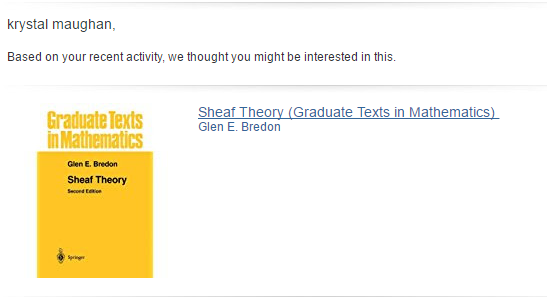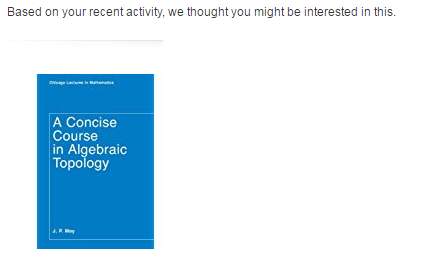## But since programming is ALL about screwing up…haha

• I “don’t read”, so I didn’t put the files into a folder and used a new container for my Firebase branch test that wasn’t synched properly.

Whoops!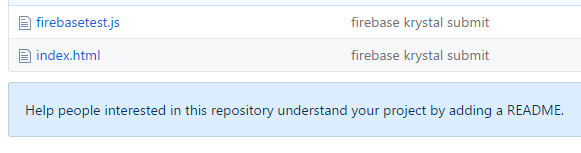## But…

• I fixed it..woohoo! Just put it on a different branch.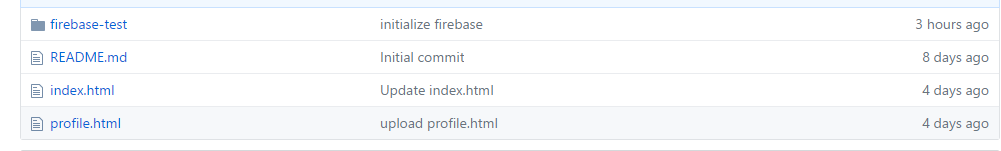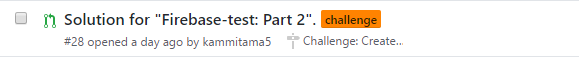## Mini-Exam

• I have a mini-exam today.

## Katas

I solved two.

• The first is: Detect if a string starts with “Can you Explain” (case-sensitive)
``````function detect(comment)
{
var word = comment.split(" ");
if ((word == "Can") && (word == "someone")
&& (word == "explain"))
{
return(true);
}
else
{
return(false);
}

}

``````

## This one I sort of duct-taped the answer, but it passed.

2 * x + another_value = total_value. Find x.

``````function twiceOfANumber(added_value, total_value) {
if ((added_value == 1) && (total_value == 2)){
return 0.5;
}
else if ((added_value == false) || (total_value == false)){
return null;
}
else if ((added_value == true) || (total_value == true)){
return null;
}

else{
var x = (total_value - (added_value)) / 2.0;
return x;
}

}
``````

## One last one…(Code Wars is so addictive!)

• This one just had sums for letters that were one side vs the other. Based on that you printed a response.

• I know..my code is too long. Lazy coder! LOL. Lazy coder whose tests passed! (haha!)
``````function alphabetWar(fight)
{
var left = 0;
var right = 0;
for (var i = 0; i < fight.length; i++){
if (fight[i] == 'w'){
left = left + 4;
}
else if (fight[i] == 'p')
{
left = left + 3;
}
else if (fight[i] == 'b')
{
left = left + 2;
}
else if (fight[i] == 's'){
left = left + 1;
}
else if (fight[i] == 'm'){
right = right + 4;
}
else if (fight[i] == 'q'){
right = right + 3;
}
else if (fight[i] == 'd'){
right = right + 2;
}
else if (fight[i] == 'z'){
right = right + 1;
}

}
if (right == left){
return("Let's fight again!")
}
else if (right > left){
return("Right side wins!")
}
else{
return("Left side wins!")
}
}
``````

## Plan for today

• Review C++ notes for mini-exam.

• Start Algorithms coursework

• If I have time, look at Firebase implementation (since we’ll be using that this Saturday).

## Update…and Finally…

• A huge application part-y thingy was approved for my green card. So, looks like you are stuck with me in this beautiful country! Mwahaha! :) dances
Written on June 6, 2017